# 九、学习对象跟踪

• 如何跟踪特定颜色的对象
• 如何构建交互式对象跟踪器
• 什么是拐角探测器？
• 如何检测要跟踪的好特征
• 如何构建基于光流的特征跟踪器

# 跟踪特定颜色的对象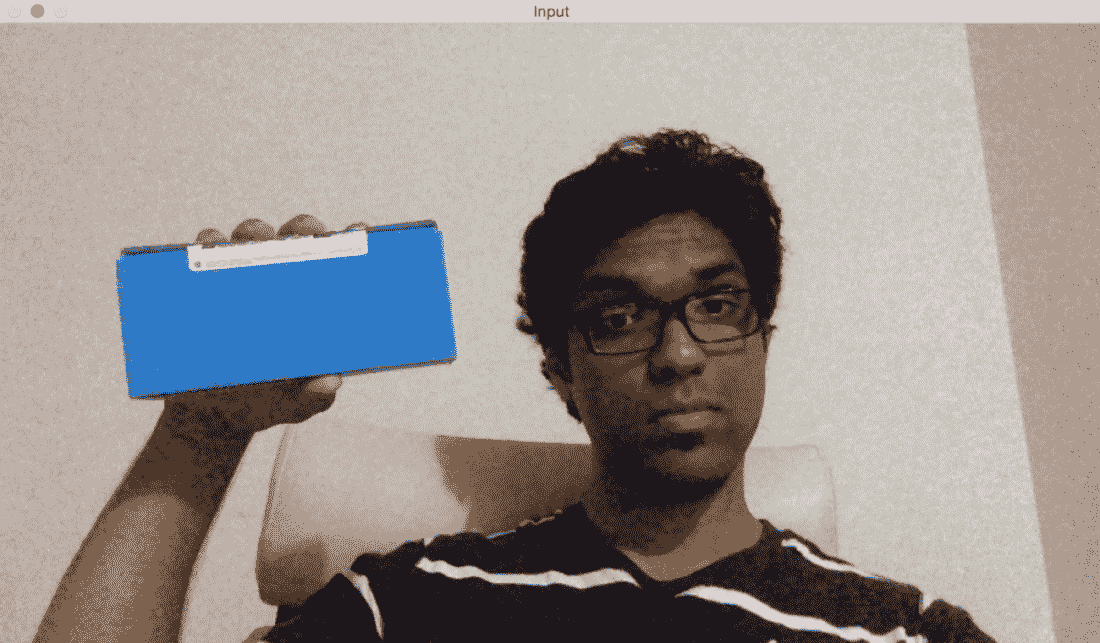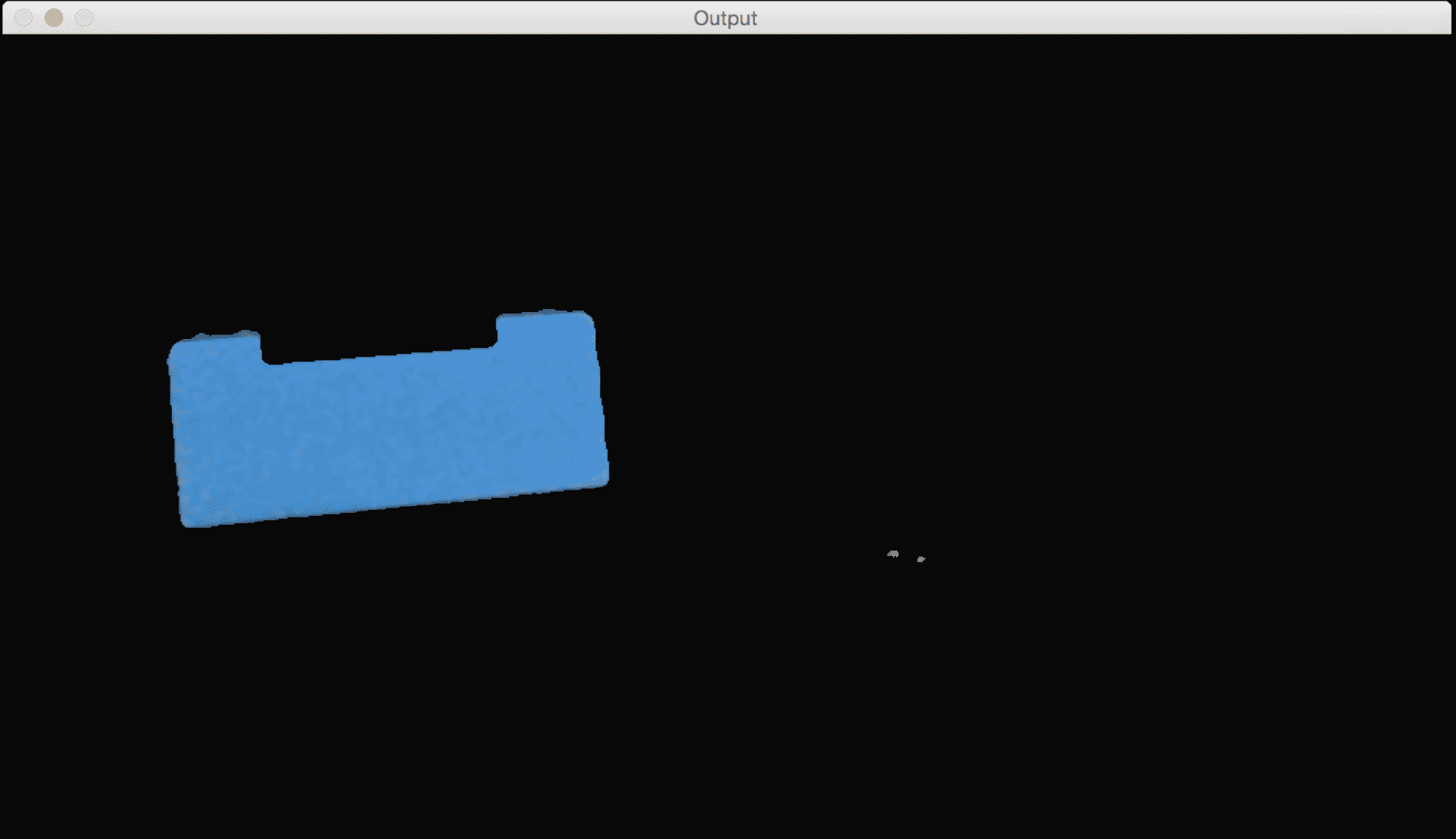int main(int argc, char* argv[])
{
// Variable declarations and initializations

// Iterate until the user presses the Esc key
while(true)
{
// Initialize the output image before each iteration
outputImage = Scalar(0,0,0);

// Capture the current frame
cap >> frame;

// Check if 'frame' is empty
if(frame.empty())
break;

// Resize the frame
resize(frame, frame, Size(), scalingFactor, scalingFactor, INTER_AREA);

// Convert to HSV colorspace
cvtColor(frame, hsvImage, COLOR_BGR2HSV);

// Define the range of "blue" color in HSV colorspace
Scalar lowerLimit = Scalar(60,100,100);
Scalar upperLimit = Scalar(180,255,255);

// Threshold the HSV image to get only blue color

// Compute bitwise-AND of input image and mask

// Run median filter on the output to smoothen it
medianBlur(outputImage, outputImage, 5);

// Display the input and output image
imshow("Input", frame);
imshow("Output", outputImage);

// Get the keyboard input and check if it's 'Esc'
// 30 -> wait for 30 ms
// 27 -> ASCII value of 'ESC' key
ch = waitKey(30);
if (ch == 27) {
break;
}
}

return 1;
}


# 构建交互式对象跟踪器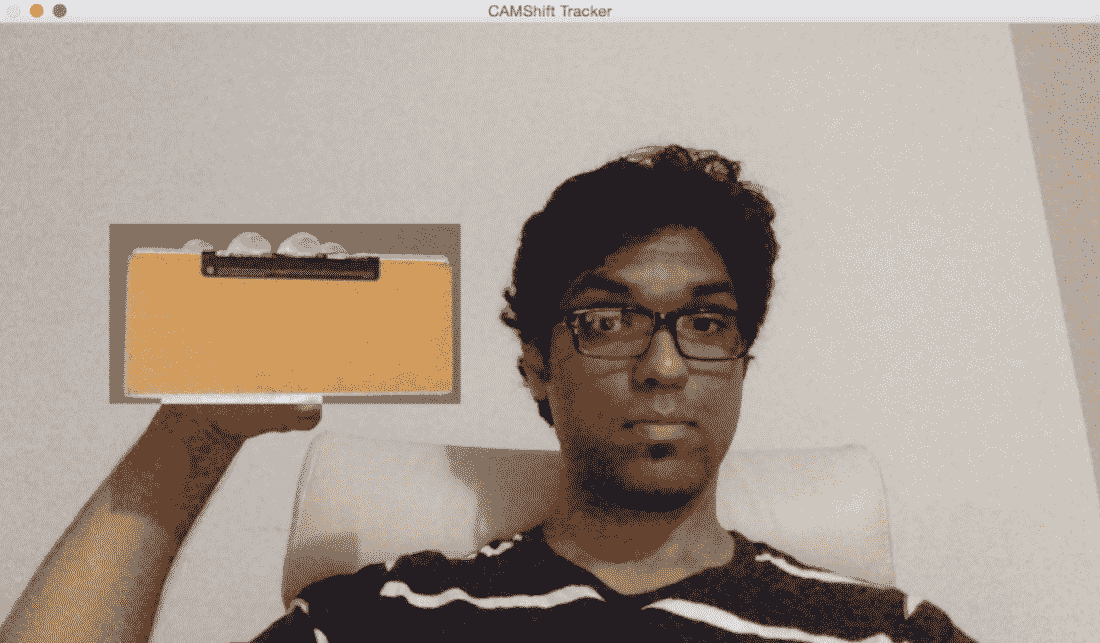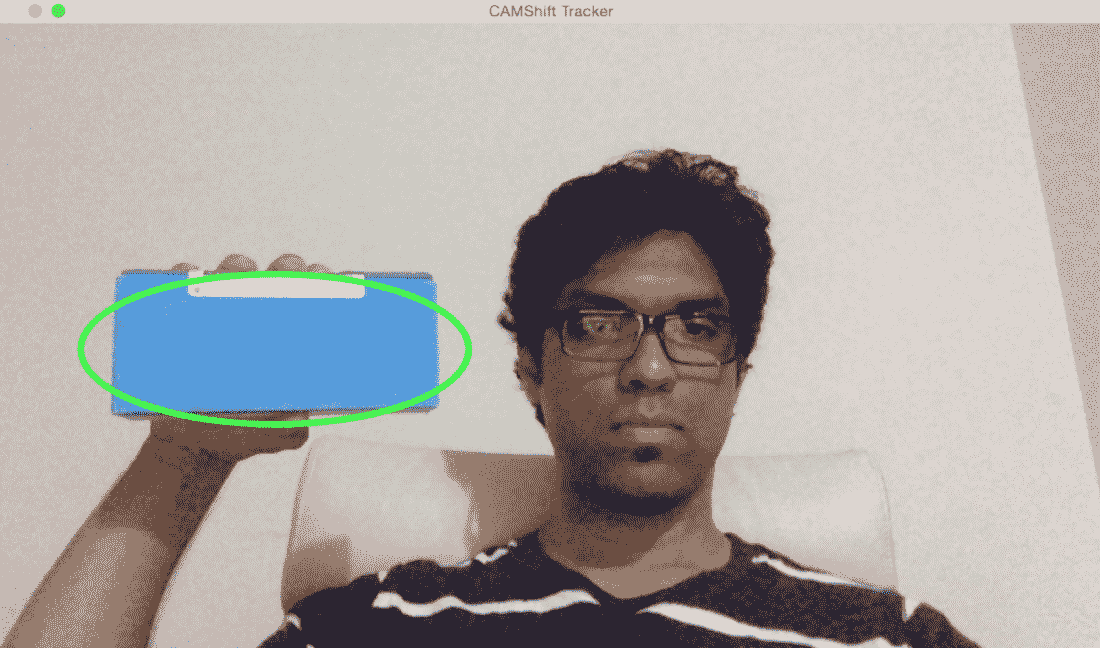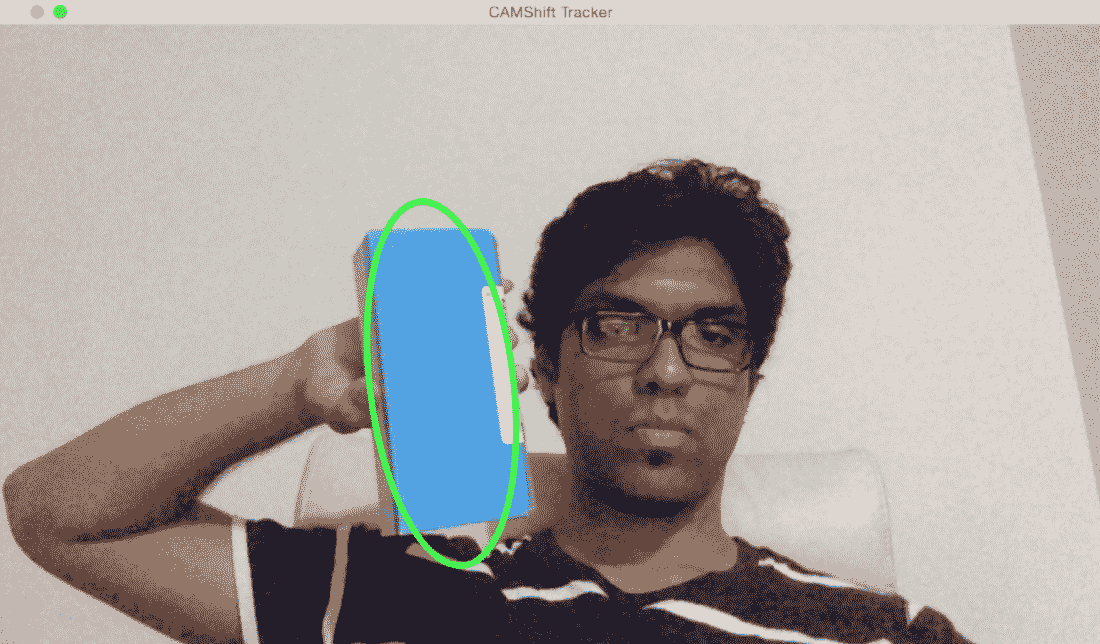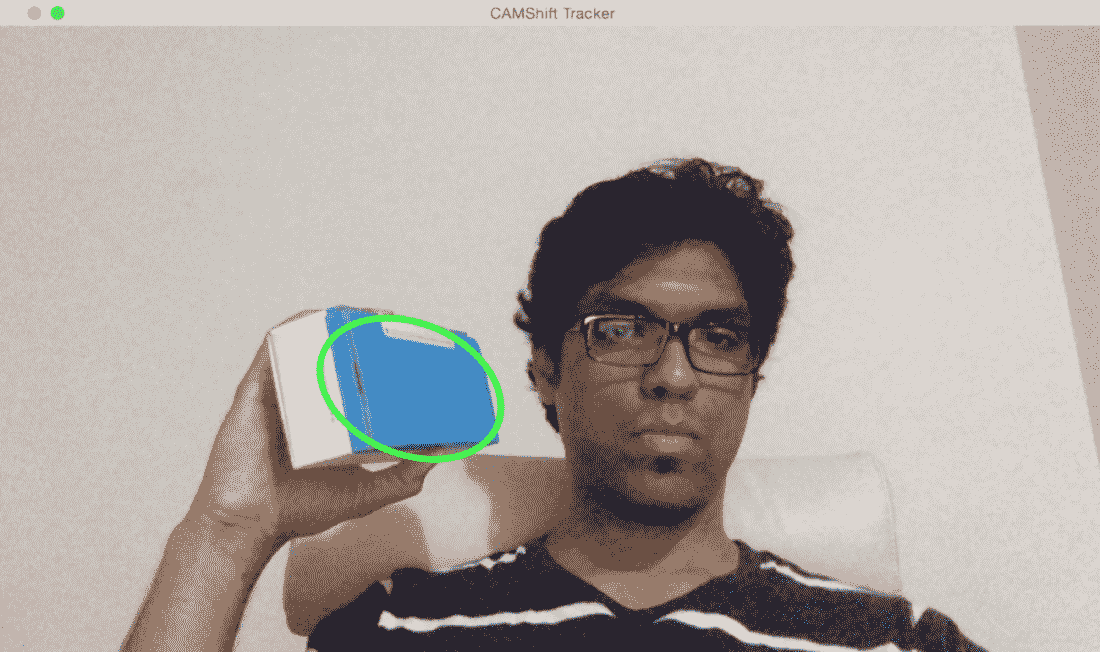Mat image;
Point originPoint;
Rect selectedRect;
bool selectRegion = false;
int trackingFlag = 0;

// Function to track the mouse events
void onMouse(int event, int x, int y, int, void*)
{
if(selectRegion)
{
selectedRect.x = MIN(x, originPoint.x);
selectedRect.y = MIN(y, originPoint.y);
selectedRect.width = std::abs(x - originPoint.x);
selectedRect.height = std::abs(y - originPoint.y);

selectedRect &= Rect(0, 0, image.cols, image.rows);
}

switch(event)
{
case EVENT_LBUTTONDOWN:
originPoint = Point(x,y);
selectedRect = Rect(x,y,0,0);
selectRegion = true;
break;

case EVENT_LBUTTONUP:
selectRegion = false;
if( selectedRect.width > 0 && selectedRect.height > 0 )
{
trackingFlag = -1;
}
break;
}
}


int main(int argc, char* argv[])
{
// Variable declaration and initialization
....
// Iterate until the user presses the Esc key
while(true)
{
// Capture the current frame
cap >> frame;

// Check if 'frame' is empty
if(frame.empty())
break;

// Resize the frame
resize(frame, frame, Size(), scalingFactor, scalingFactor, INTER_AREA);

// Clone the input frame
frame.copyTo(image);

// Convert to HSV colorspace
cvtColor(image, hsvImage, COLOR_BGR2HSV);


        if(trackingFlag)
{
// Check for all the values in 'hsvimage' that are within the specified range
// and put the result in 'mask'
inRange(hsvImage, Scalar(0, minSaturation, minValue), Scalar(180, 256, maxValue), mask);

// Mix the specified channels
int channels[] = {0, 0};
hueImage.create(hsvImage.size(), hsvImage.depth());
mixChannels(&hsvImage, 1, &hueImage, 1, channels, 1);

if(trackingFlag < 0)
{
// Create images based on selected regions of interest

// Compute the histogram and normalize it
calcHist(&roi, 1, 0, maskroi, hist, 1, &histSize, &histRanges);
normalize(hist, hist, 0, 255, NORM_MINMAX);

trackingRect = selectedRect;
trackingFlag = 1;
}


            // Compute the histogram backprojection
calcBackProject(&hueImage, 1, 0, hist, backproj, &histRanges);
RotatedRect rotatedTrackingRect = CamShift(backproj, trackingRect, TermCriteria(TermCriteria::EPS | TermCriteria::COUNT, 10, 1));

// Check if the area of trackingRect is too small
if(trackingRect.area() <= 1)
{
// Use an offset value to make sure the trackingRect has a minimum size
int cols = backproj.cols, rows = backproj.rows;
int offset = MIN(rows, cols) + 1;
trackingRect = Rect(trackingRect.x - offset, trackingRect.y - offset, trackingRect.x + offset, trackingRect.y + offset) & Rect(0, 0, cols, rows);
}


            // Draw the ellipse on top of the image
ellipse(image, rotatedTrackingRect, Scalar(0,255,0), 3, LINE_AA);
}

// Apply the 'negative' effect on the selected region of interest
if(selectRegion && selectedRect.width > 0 && selectedRect.height > 0)
{
Mat roi(image, selectedRect);
bitwise_not(roi, roi);
}

// Display the output image
imshow(windowName, image);

// Get the keyboard input and check if it's 'Esc'
// 27 -> ASCII value of 'Esc' key
ch = waitKey(30);
if (ch == 27) {
break;
}
}

return 1;
}


# 使用 Harris 角点检测器检测点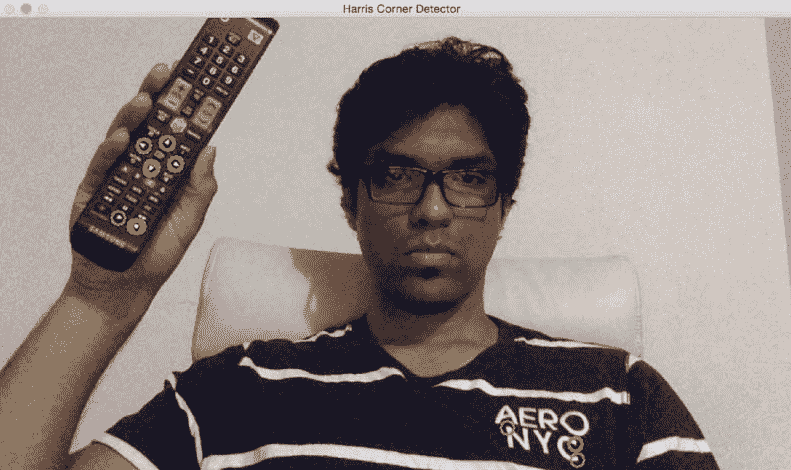int main(int argc, char* argv[])
{
// Variable declaration and initialization

// Iterate until the user presses the Esc key
while(true)
{
// Capture the current frame
cap >> frame;

// Resize the frame
resize(frame, frame, Size(), scalingFactor, scalingFactor, INTER_AREA);

dst = Mat::zeros(frame.size(), CV_32FC1);

// Convert to grayscale
cvtColor(frame, frameGray, COLOR_BGR2GRAY );

// Detecting corners
cornerHarris(frameGray, dst, blockSize, apertureSize, k, BORDER_DEFAULT);

// Normalizing
normalize(dst, dst_norm, 0, 255, NORM_MINMAX, CV_32FC1, Mat());
convertScaleAbs(dst_norm, dst_norm_scaled);


        // Drawing a circle around each corner
for(int j = 0; j < dst_norm.rows ; j++)
{
for(int i = 0; i < dst_norm.cols; i++)
{
if((int)dst_norm.at<float>(j,i) > thresh)
{
circle(frame, Point(i, j), 8, Scalar(0,255,0), 2, 8, 0);
}
}
}

// Showing the result
imshow(windowName, frame);

// Get the keyboard input and check if it's 'Esc'
// 27 -> ASCII value of 'Esc' key
ch = waitKey(10);
if (ch == 27) {
break;
}
}

// Release the video capture object
cap.release();

// Close all windows
destroyAllWindows();

return 1;
}


# 要跟踪的良好功能

Harris 角点检测器在很多情况下都表现良好，但仍有改进的余地。 大约在HarrisStephensShiiTomasi最初的论文发表 6 年后，他们将其称为跟踪的良好特征。 你可以在这里阅读原文：http://www.ai.mit.edu/courses/6.891/handouts/shi94good.pdf。 他们使用了不同的评分函数来提高整体质量。 使用这种方法，我们可以在给定的图像中找到 N 个最强的角点。 当我们不想使用每个角落来从图像中提取信息时，这是非常有用的。 正如我们所讨论的，一个好的兴趣点检测器在目标跟踪、目标识别和图像搜索等应用中非常有用。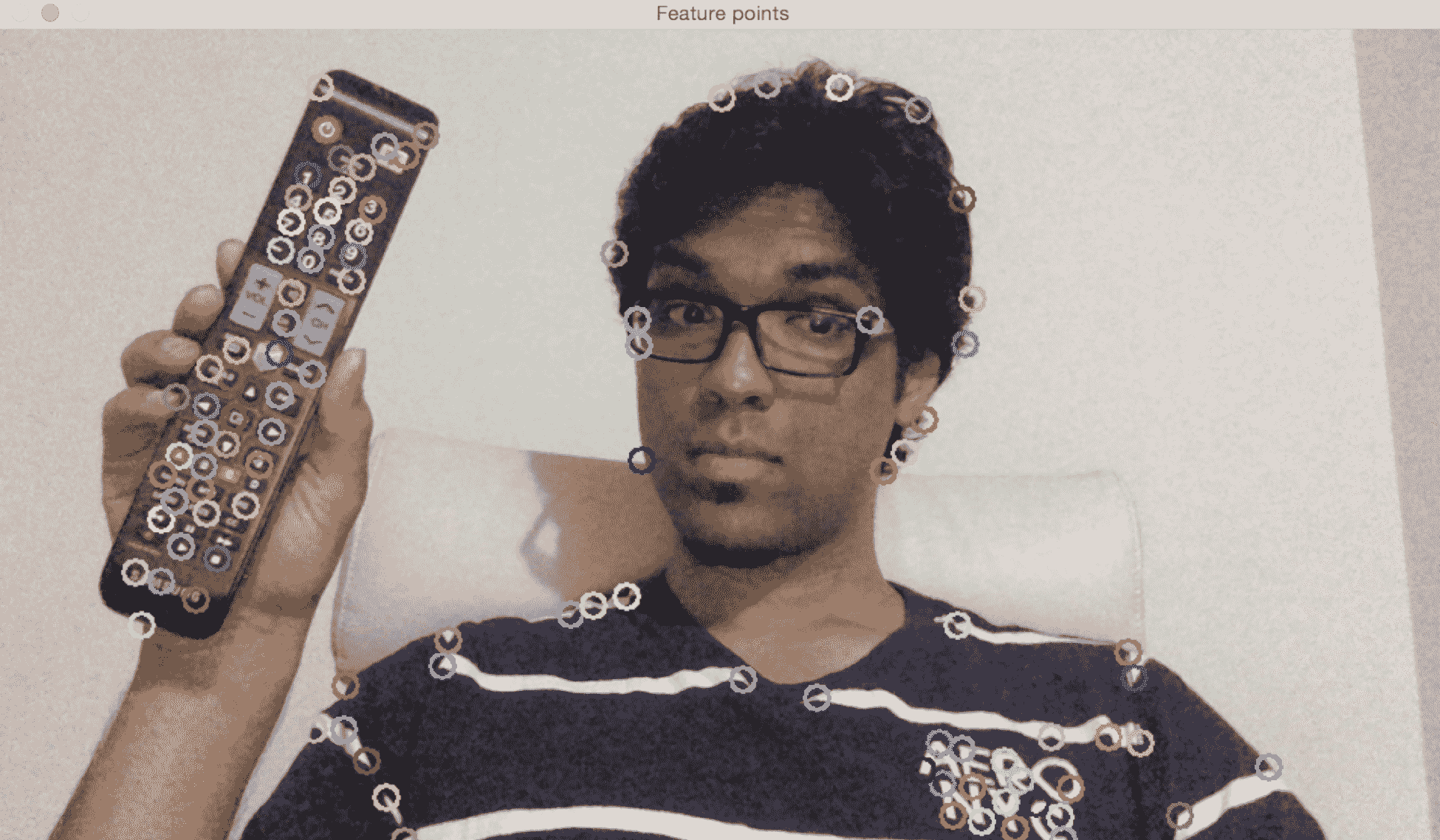int main(int argc, char* argv[])
{
// Variable declaration and initialization

// Iterate until the user presses the Esc key
while(true)
{
// Capture the current frame
cap >> frame;

// Resize the frame
resize(frame, frame, Size(), scalingFactor, scalingFactor, INTER_AREA);

// Convert to grayscale
cvtColor(frame, frameGray, COLOR_BGR2GRAY );

// Initialize the parameters for Shi-Tomasi algorithm
vector<Point2f> corners;
double qualityThreshold = 0.02;
double minDist = 15;
int blockSize = 5;
bool useHarrisDetector = false;
double k = 0.07;

// Clone the input frame
Mat frameCopy;
frameCopy = frame.clone();

// Apply corner detection
goodFeaturesToTrack(frameGray, corners, numCorners, qualityThreshold, minDist, Mat(), blockSize, useHarrisDetector, k);


        // Parameters for the circles to display the corners
int thickness = 2;   // thickness of the circles
int lineType = 8;

// Draw the detected corners using circles
for(size_t i = 0; i < corners.size(); i++)
{
Scalar color = Scalar(rng.uniform(0,255), rng.uniform(0,255), rng.uniform(0,255));
circle(frameCopy, corners[i], radius, color, thickness, lineType, 0);
}

/// Show what you got
imshow(windowName, frameCopy);

// Get the keyboard input and check if it's 'Esc'
// 27 -> ASCII value of 'Esc' key
ch = waitKey(30);
if (ch == 27) {
break;
}
}

// Release the video capture object
cap.release();

// Close all windows
destroyAllWindows();

return 1;
}


# 卢卡斯-卡纳德法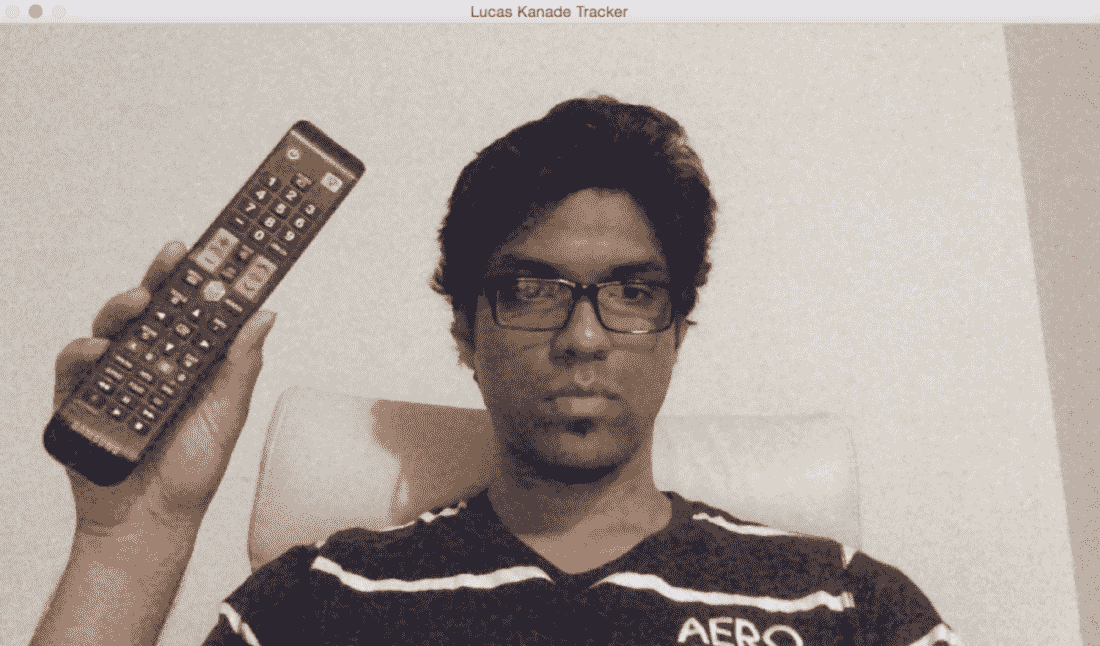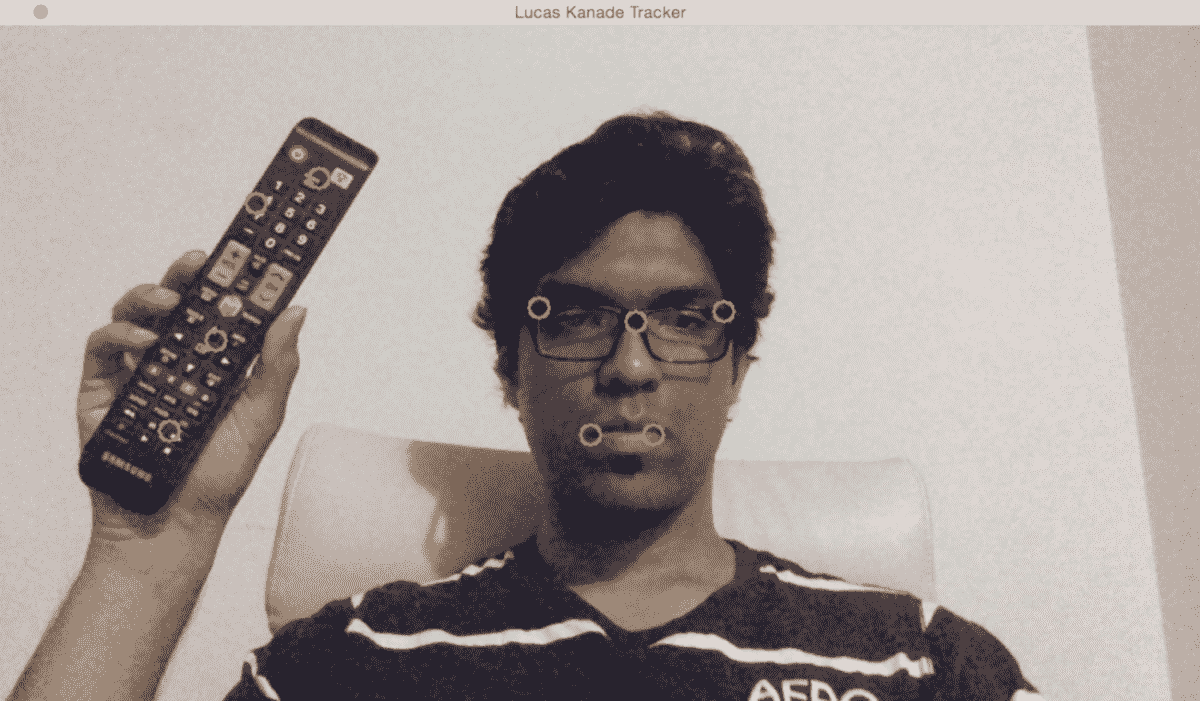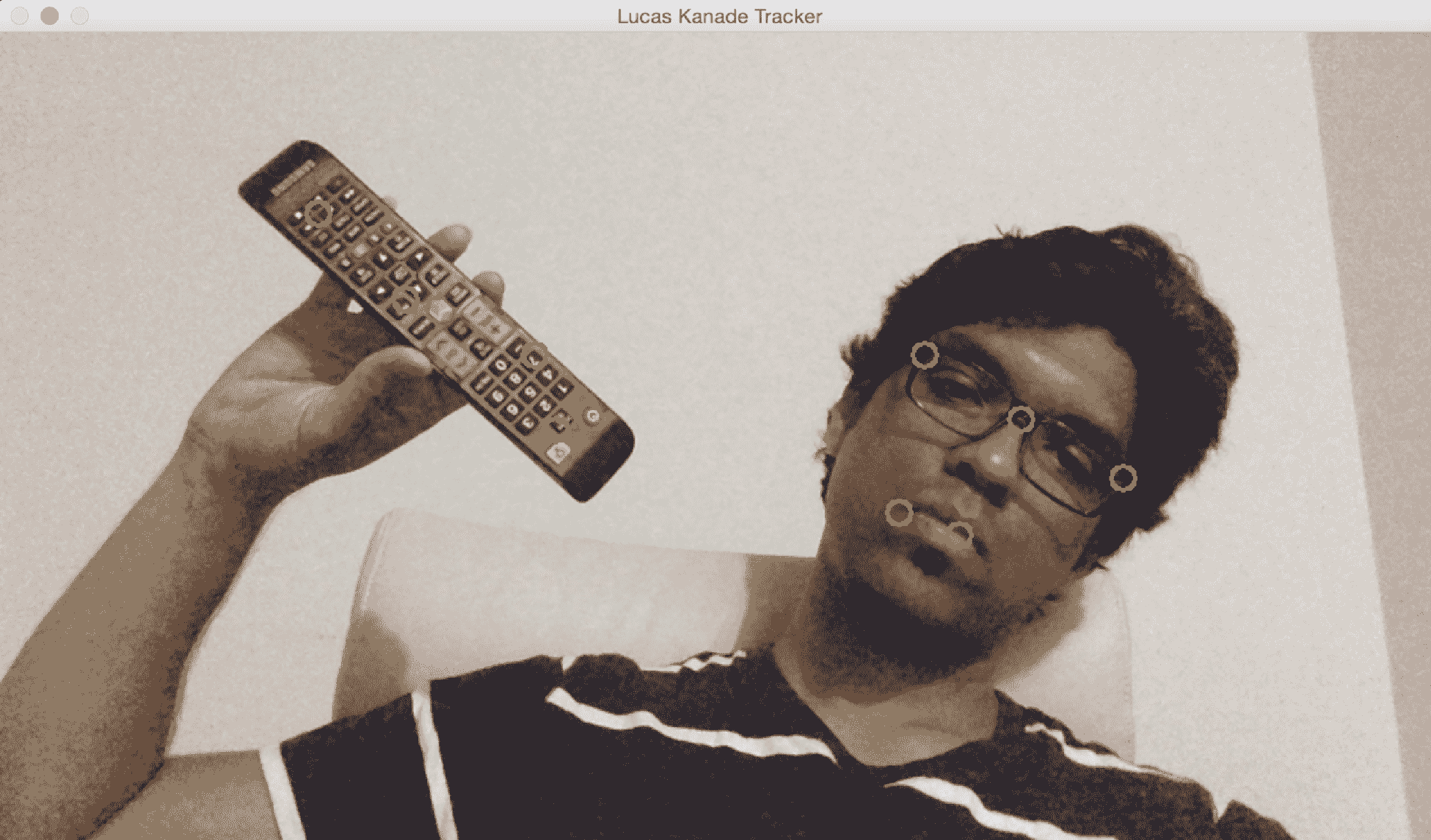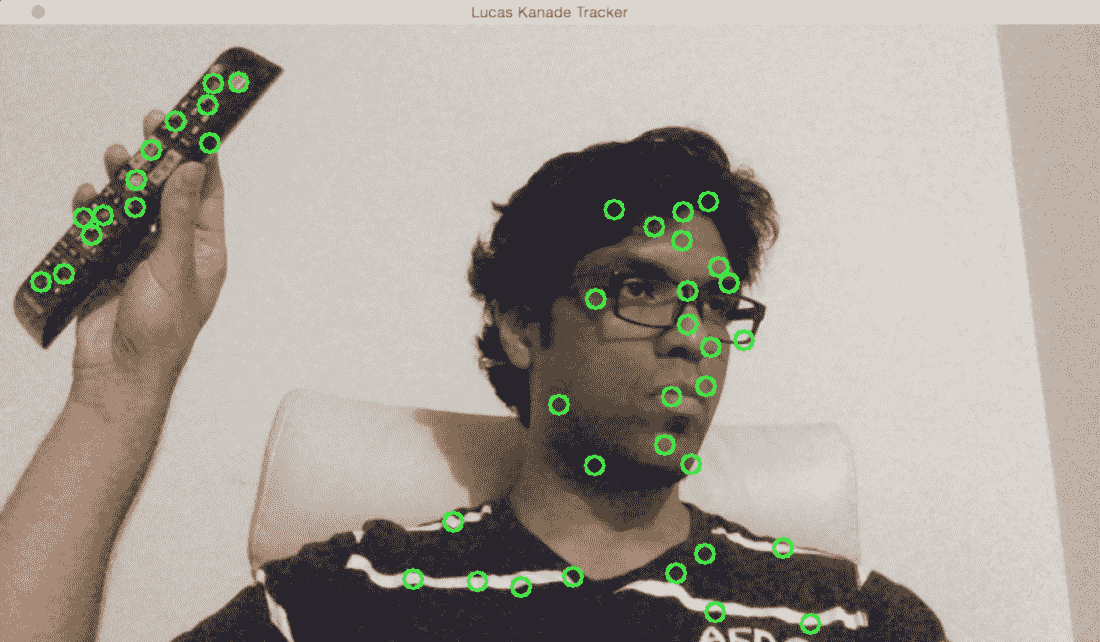int main(int argc, char* argv[])
{
// Variable declaration and initialization

// Iterate until the user hits the Esc key
while(true)
{
// Capture the current frame
cap >> frame;

// Check if the frame is empty
if(frame.empty())
break;

// Resize the frame
resize(frame, frame, Size(), scalingFactor, scalingFactor, INTER_AREA);

// Copy the input frame
frame.copyTo(image);

// Convert the image to grayscale
cvtColor(image, curGrayImage, COLOR_BGR2GRAY);

// Check if there are points to track
if(!trackingPoints.empty())
{
// Status vector to indicate whether the flow for the corresponding features has been found
vector<uchar> statusVector;

// Error vector to indicate the error for the corresponding feature
vector<float> errorVector;

// Check if previous image is empty
if(prevGrayImage.empty())
{
curGrayImage.copyTo(prevGrayImage);
}

// Calculate the optical flow using Lucas-Kanade algorithm
calcOpticalFlowPyrLK(prevGrayImage, curGrayImage, trackingPoints, trackingPoints, statusVector, errorVector, windowSize, 3, terminationCriteria, 0, 0.001);



int count = 0;

// Minimum distance between any two tracking points
int minDist = 7;

for(int i=0; i < trackingPoints.size(); i++)
{
if(pointTrackingFlag)
{
// If the new point is within 'minDist' distance from an existing point, it will not be tracked
if(norm(currentPoint - trackingPoints[i]) <= minDist)
{
pointTrackingFlag = false;
continue;
}
}

// Check if the status vector is good
if(!statusVector[i])
continue;

trackingPoints[count++ ] = trackingPoints[i];

// Draw a filled circle for each of the tracking points
int thickness = 2;
int lineType = 8;
circle(image, trackingPoints[i], radius, Scalar(0,255,0), thickness, lineType);
}

trackingPoints.resize(count);
}



// Refining the location of the feature points
if(pointTrackingFlag && trackingPoints.size() < maxNumPoints)
{
vector<Point2f> tempPoints;
tempPoints.push_back(currentPoint);

// Function to refine the location of the corners to subpixel accuracy.
// Here, 'pixel' refers to the image patch of size 'windowSize' and not the actual image pixel
cornerSubPix(curGrayImage, tempPoints, windowSize, Size(-1,-1), terminationCriteria);

trackingPoints.push_back(tempPoints);
pointTrackingFlag = false;
}

// Display the image with the tracking points
imshow(windowName, image);

// Check if the user pressed the Esc key
char ch = waitKey(10);
if(ch == 27)
break;

// Swap the 'points' vectors to update 'previous' to 'current'
std::swap(trackingPoints, trackingPoints);

// Swap the images to update previous image to current image
cv::swap(prevGrayImage, curGrayImage);
}

return 1;
}


# Farneback 算法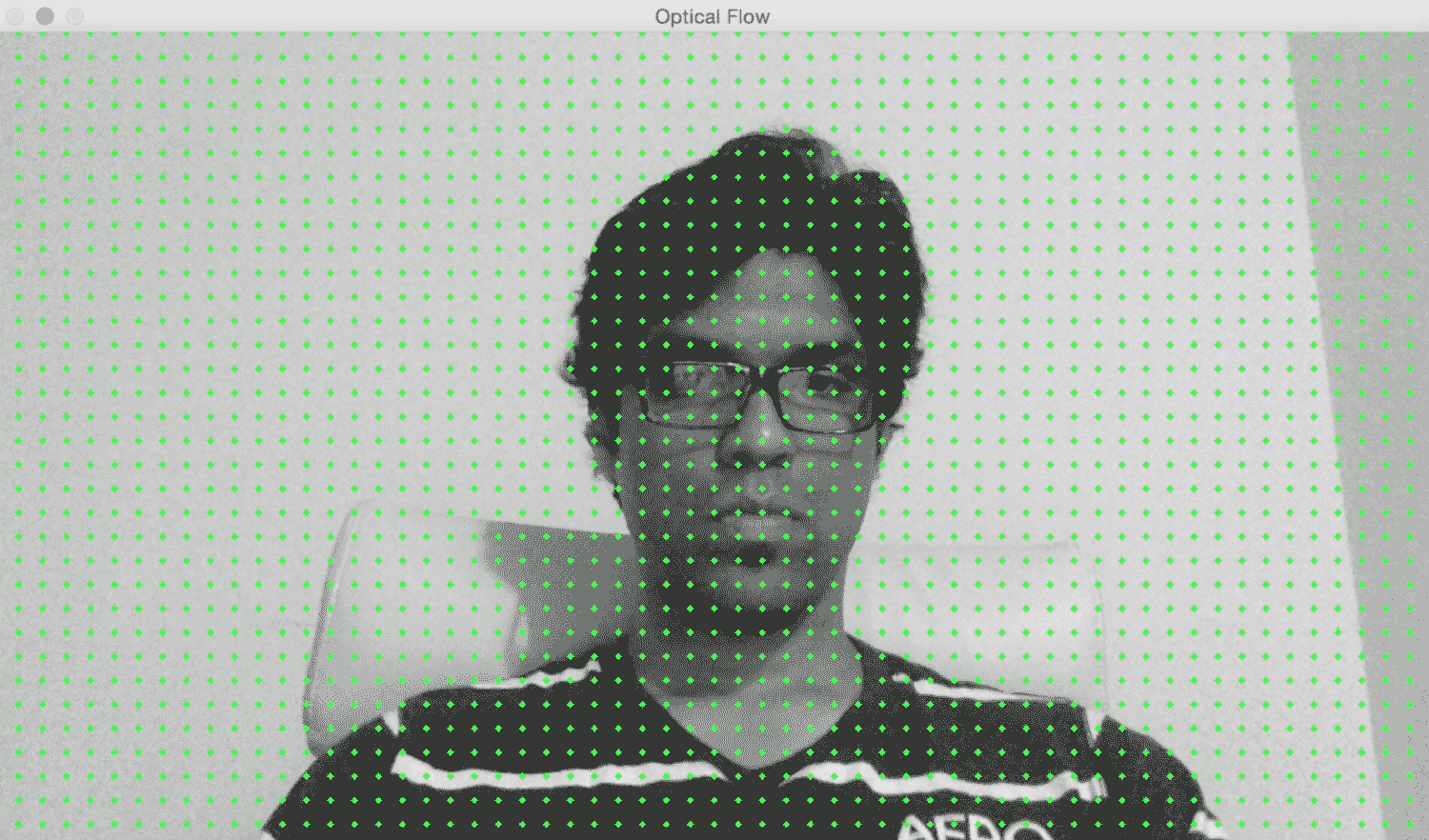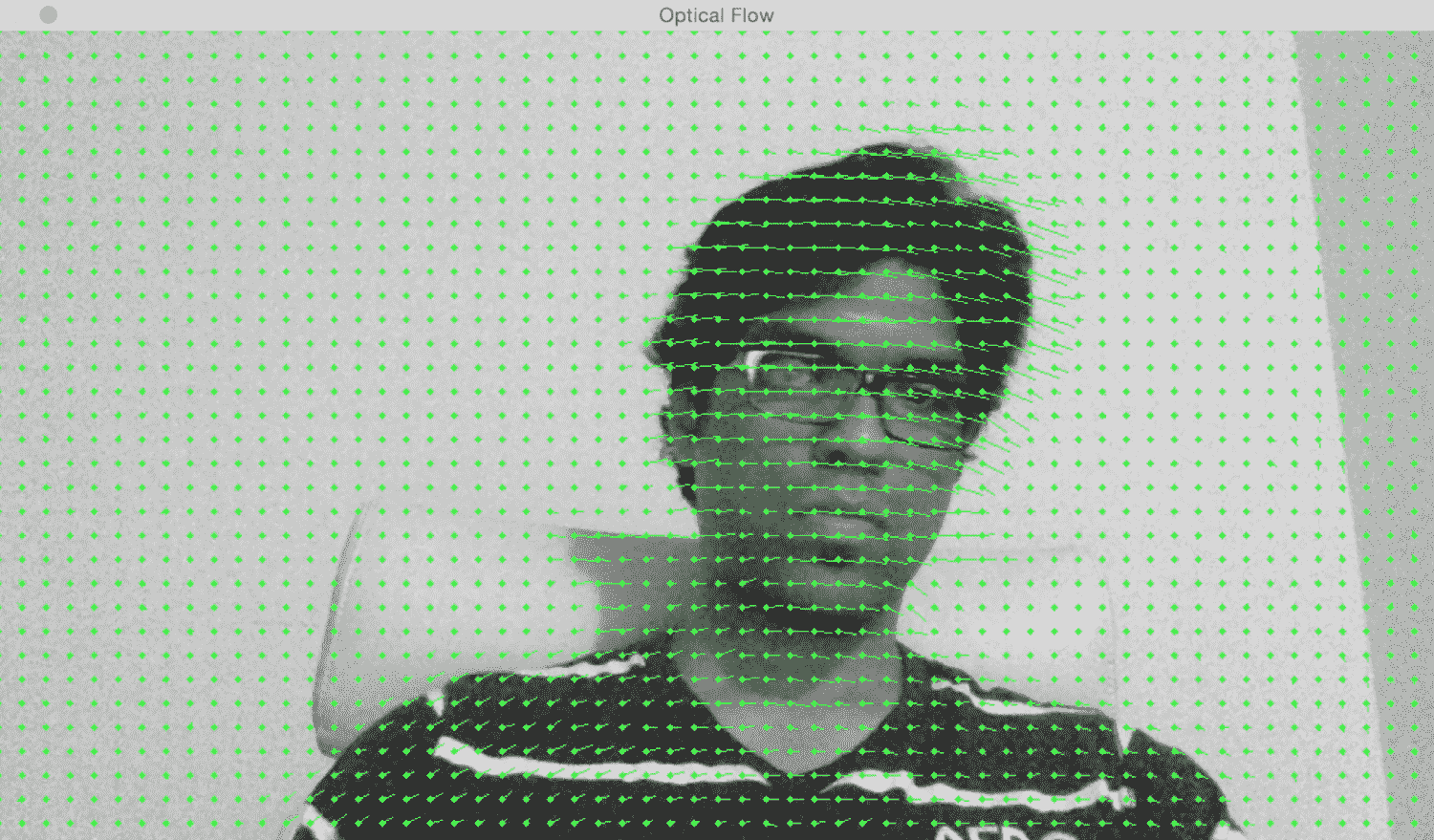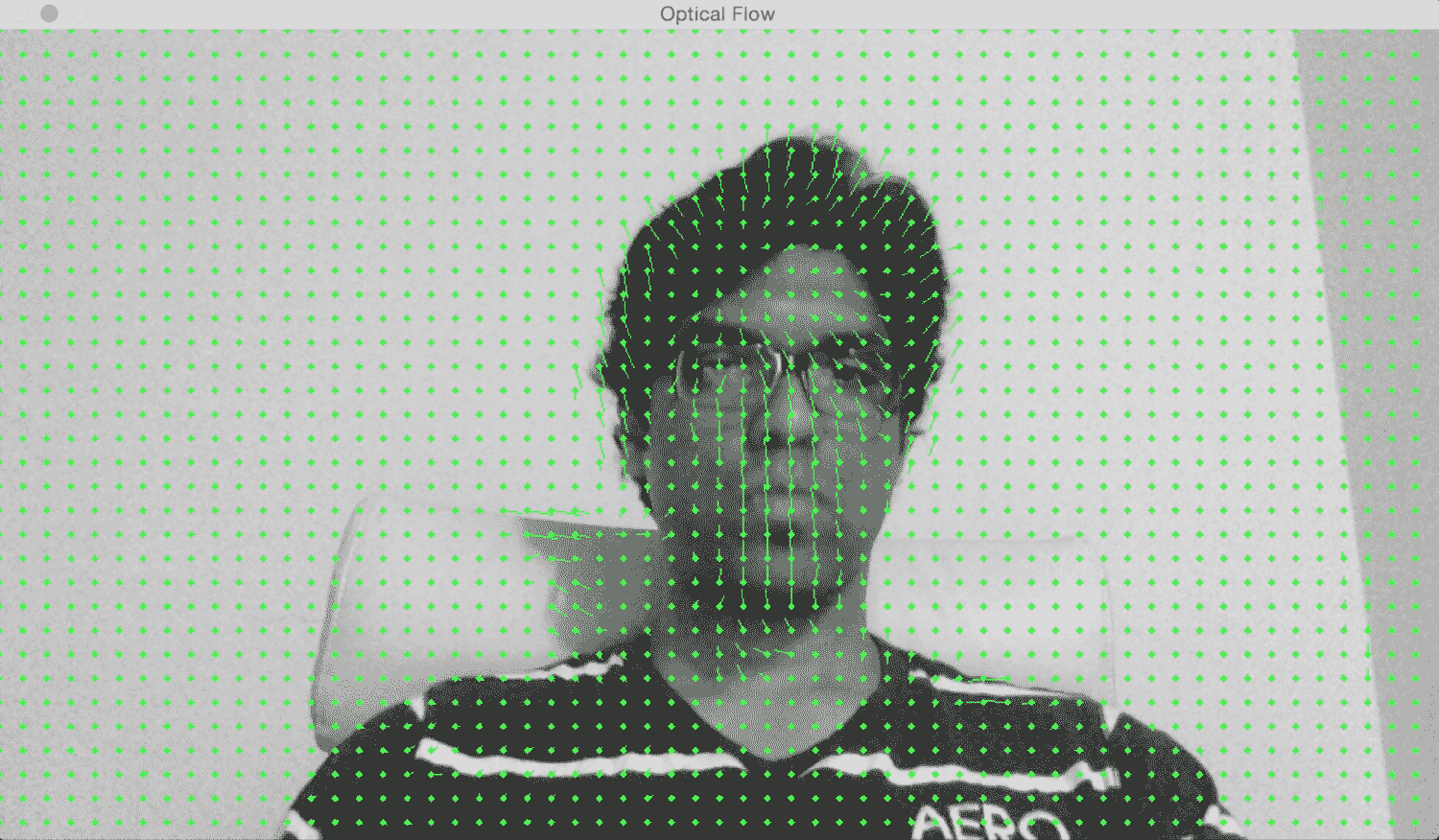int main(int, char** argv)
{
// Variable declaration and initialization

// Iterate until the user presses the Esc key
while(true)
{
// Capture the current frame
cap >> frame;

if(frame.empty())
break;

// Resize the frame
resize(frame, frame, Size(), scalingFactor, scalingFactor, INTER_AREA);

// Convert to grayscale
cvtColor(frame, curGray, COLOR_BGR2GRAY);

// Check if the image is valid
if(prevGray.data)
{
// Initialize parameters for the optical flow algorithm
float pyrScale = 0.5;
int numLevels = 3;
int windowSize = 15;
int numIterations = 3;
int neighborhoodSize = 5;
float stdDeviation = 1.2;

// Calculate optical flow map using Farneback algorithm
calcOpticalFlowFarneback(prevGray, curGray, flowImage, pyrScale, numLevels, windowSize, numIterations, neighborhoodSize, stdDeviation, OPTFLOW_USE_INITIAL_FLOW);


            // Convert to 3-channel RGB
cvtColor(prevGray, flowImageGray, COLOR_GRAY2BGR);

// Draw the optical flow map
drawOpticalFlow(flowImage, flowImageGray);

// Display the output image
imshow(windowName, flowImageGray);
}

// Break out of the loop if the user presses the Esc key
ch = waitKey(10);
if(ch == 27)
break;

// Swap previous image with the current image
std::swap(prevGray, curGray);
}

return 1;
}


// Function to compute the optical flow map
void drawOpticalFlow(const Mat& flowImage, Mat& flowImageGray)
{
int stepSize = 16;
Scalar color = Scalar(0, 255, 0);

// Draw the uniform grid of points on the input image along with the motion vectors
for(int y = 0; y < flowImageGray.rows; y += stepSize)
{
for(int x = 0; x < flowImageGray.cols; x += stepSize)
{
// Circles to indicate the uniform grid of points
int thickness = -1;

// Lines to indicate the motion vectors
Point2f pt = flowImage.at<Point2f>(y, x);
line(flowImageGray, Point(x,y), Point(cvRound(x+pt.x), cvRound(y+pt.y)), color);
}
}
}